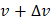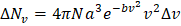# The distribution of the molecular velocities of gas molecules at any temperatureis shown below. (The plot below is known as Maxwell’s distribution of molecular speeds.)Whereis molecular velocityis number of molecules having velocityLet us define, which is equal to the number of molecules between the velocity rangeand, given byWhereis total number of moleculesandis universal gas constantis temperature of the gasis molecular weight of the gas SI units ofare a)b)c)d)## Question ID - 103080 :- The distribution of the molecular velocities of gas molecules at any temperatureis shown below. (The plot below is known as Maxwell’s distribution of molecular speeds.)Whereis molecular velocityis number of molecules having velocityLet us define, which is equal to the number of molecules between the velocity rangeand, given byWhereis total number of moleculesandis universal gas constantis temperature of the gasis molecular weight of the gas SI units ofare a)b)c)d)3537

 (b)Next Question :

Two flasksandhave equal volume.is maintained at 300 K andat 600 K. Whilecontainsgas,has an equal mass ofgas. Assuming ideal behaviours for both the gases, answer the following:

 Flask containing greater number of molecules a)b)c) Bothandd) None